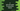# C program to print all uppercase and lowercase letters in the alphabet### Write a C program to print all Uppercase and Lowercase letters in the alphabet:

In this example program, we will learn how to print the uppercase and lowercase letters in C programming language. We will use one for loop to print the characters. Let’s take a look into the program :

### C program :

``````#include

int main()
{
//1
char ch;

//2
printf("Uppercase characters : \n");
//3
for(ch = 'A';ch <= 'Z';ch++){
printf("%c ",ch);
}

//4
printf("\nLowercase characters : \n");
for(ch = 'a';ch <= 'z';ch++){
printf("%c ",ch);
}

return 0;
}``````

### Explanation :

The commented numbers in the above program denotes the step number below :

1. Create one character variable ‘ch’.
2. Start printing the uppercase characters.
3. Start a for loop. Start the loop from ch = ‘A’ to ch = ‘Z’. And in the loop, increment the value of ch by 1. i.e. we will increase the ASCII value for that character . In the loop, print the character value or the value of ch.
4. Similarly, print all lowercase letters.

### Output of the program :

``````Uppercase characters :
A B C D E F G H I J K L M N O P Q R S T U V W X Y Z
Lowercase characters :
a b c d e f g h i j k l m n o p q r s t u v w x y z``````